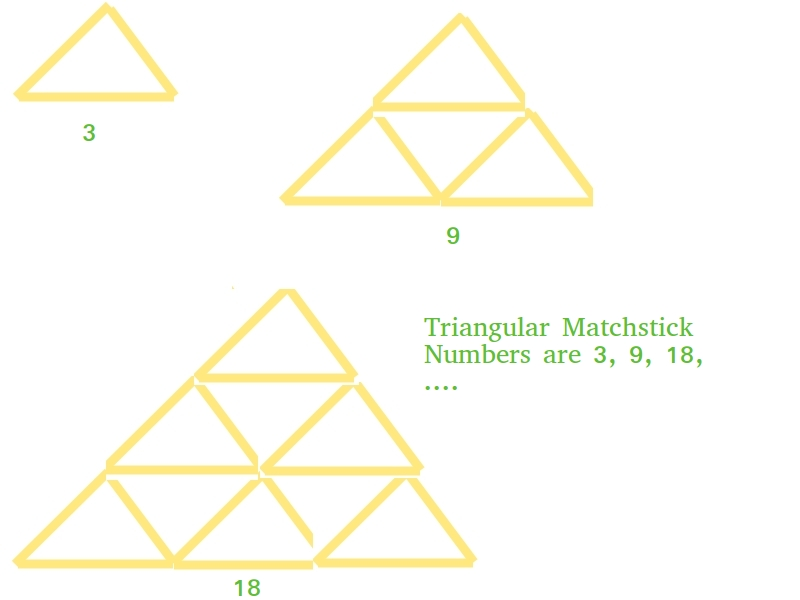# Python Program for Triangular Matchstick Number

python tutorials and learn python

# Python Program for Triangular Matchstick Number

Given a number X which represents the floor of a matchstick pyramid, write a program to print the total number of matchstick required to form a pyramid of matchsticks of x floors.

Examples:

```Input : X = 1
Output : 3
Input : X = 2
Output : 9
```

This is mainly an extension of triangular numbers. For a number X, the matchstick required will be three times of X-th triangular numbers, i.e., (3*X*(X+1))/2`# Python program to find X-th triangular ``# matchstick number `` ``def` `numberOfSticks(x): ``    ``return` `(``3` `*` `x ``*` `(x ``+` `1``)) ``/` `2``     ``# main() ``print``(``int``(numberOfSticks(``7``))) `
Output:

`84`